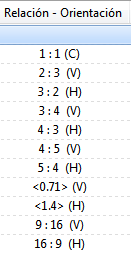# Aspect Ratio column

The Aspect Ratio column shows the ratio of the division between the number of horizontal and vertical pixels (1.78, 1, etc.), but there will be some way for this column or some other column to display the native aspect ratio, for example, 16:9, 4:3. 1:1?

yes, try this

2 Likes

Thank you very much @fkast, excellent!

If you group by that column, it should bucket things similarly. We'll be expanding this soon to make the same bucketing available as an actual column, as well as expanding the number/types of buckets.

I grouped the files based on the Aspect Ratio column, but the result remains the same. Thank you very much @Leo, both for the response and for the improvements you plan to make to this column!

Here is exactly what you want
Ratio.js.txt (1.4 KB)
remove the `.txt` extension and drag/install in scripts .

remember an image widthxheight that is 3840x2160 will reduce to a ratio of 16:9
but an image 3841x2160 will not, it will show as 3841:2160

Thank you very much @galaxyhub, perfect, all your scripts are very useful!Why is it that for some images the displayed aspect ratio is such a large number? For example, I have a Polaroid image with a resolution of 1182 x 946 pixels and an aspect ratio according to Photoshop of 5:4, yet the script shows me 591:473? The greatest common factor is being calculated well, in this case it is 2.

1182 / `2` = 591
946 / `2` = 473
The `Ratio` column is exact, the `Aspect` column is less exact and is probably similar to what photoshop is doing. This is why I called it `Aspect` and not `Ratio` or `Aspect Ratio`.

I understand, thank you very much!

Adapting the script from @galaxyhub, and recommended by @kfast, I obtained the following code, which allows creating a column to display the most important aspect ratios (1:1, 5:4, 4:3, 3:2, 16:9 and 2.39:1), it also allows you to display the orientation of the images: V for Vertical images and H for Horizontal images.

For images with aspect ratios other than those specified, the aspect ratio is displayed in decimal form and enclosed between "<" and ">" to create some visual difference from the others.With `toFixed(2)` I get a number rounded to two decimal places, but if the second decimal place is a zero (0), it is ignored. What could I do to always show the two decimal places?

Example: 1.40

``````var ar = c.metadata.image.aspectratio; // Aspect Ratio
var arPercent = ar * 100 // Percent
var arRound = Number(ar.toFixed(2)); // Round
``````

I'm wanting to establish a condition to know if a number is an integer, but it generates the following error "The object does not accept this property or method" on the following line:

`if (Number.isInteger(arRound)) {`

Complete code:

``````var arRound = Number(ar.toFixed(2));

if (Number.isInteger(arRound)) {
arRound = arRound;
} else {
arRound = (arRound + "0").slice(0, 4);
}
``````

`isInteger` is not supported.
JScript is not JavaScript. From wikipedia :

On the JavaScript front, Microsoft reverse-engineered the Navigator interpreter to create its own, called JScript.

That was 1995, `JScript` is not currently being maintained by Microsoft, it switched to `JavaScript` around 2009.

change
`var arRound = Number(ar.toFixed(2));`
to
`var arRound = ar.toFixed(2);`

How interesting to know that, I knew that Java and JavaScript are not the same thing, but I didn't know JavaScript and JScript are different things.Then I can eliminate the word Number, better yet, so the code is simpler.

I ended up creating the condition to determine if a number is an integer as follows:

`if (arRound % 1 == 0){`

Thank you very much once again for all your help!